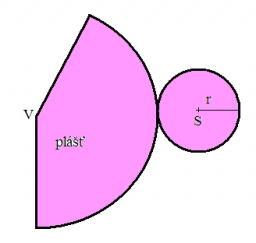# Deviation 70744

Calculate the volume and surface of the rotating cone if its height is 10 cm and the side has a deviation of 30 ° from the base plane.

V =  3141.5927 cm3
S =  2030.7574 cm2

### Step-by-step explanation:Did you find an error or inaccuracy? Feel free to write us. Thank you!

Tips for related online calculators
Need help calculating sum, simplifying, or multiplying fractions? Try our fraction calculator.
Do you know the volume and unit volume, and want to convert volume units?
The Pythagorean theorem is the base for the right triangle calculator.